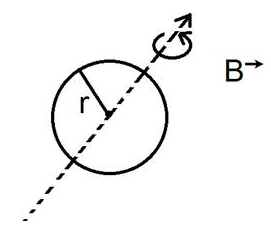# ELECTROMAGNETIC INDUCTION AND ALTERNATING CURRENTS

• Home -
• ELECTROMAGNETIC INDUCTION AND ALTERNATING CURRENTS

ELECTROMAGNETIC INDUCTION AND ALTERNATING CURRENTS - Topic Wise Multiple Choice Questions (MCQ)

Topic Wise free Electromagnetic Induction and Alternating Current MCQ with solutions for 11th 12th exam Practice Multiple Choice Questions and answer for JEE-mains State CET Engineering Entrance GATE free Online Test

1 )

A coil having area 2m2 is placed in a magnetic field which changes from 1 wb/m2 to 4 wb/m2 in an interval of 2 second. The emf induced in the coil of single turn is....

4 v
3 v
2 v
1.5 v

View Solution

2 )

Two different loops are concentric & lie in the same plane. The current in outer loop is clockwise & increasing with time. The induced current in the inner loop then, is …….

clockwise
counter clockwise
direction depends on the ratio of loop radii
zero

View Solution

3 )

A ring of radius r is rotating about its diameter with angular velocity ω in a perpendicular magnetic field B It has 20 turns. The emf induced is20Bπr^2 sin wt
20Bπr^2 cos wt
10√2Bπr^2
20Bπr^2 w sin wt

View Solution

4 )

A magnetic field 2 × 10–2T acts at right angles to a coil of area 200 cm2 with 25 turns. The average emf induced in the coil is 0.1 v when it removes from the field in time t. The value of t is

0.1 sec
1 sec
0.01 sec
1.5 sec

View Solution

5 )

The magnetic flux linked with a coil, in webers, is given by the equation ɸ = 4t2 – t + 7. Then the magnitude of induced emf at 2 sec will be ...

17 v
21 v
15 v
13 v

View Solution